# Evaluation of threading dislocation density in III-nitride epitaxial layers

## E. Dobročka

### edmund.dobrocka@savba.sk

The III–nitride semiconductors of AlN, GaN, and InN and their ternary alloys have great potential for use in optoelectronic and high-temperature electronic devices due to their wide range of bandgaps and high-temperature stability. The increasing range of their applications such as light emitting diodes, lasers and high power transistors requires the study of the influence of extended defects on the electrical and optical properties of these materials [1, 2]. The most important defects in the structures are threading dislocations (TDs). Their total density in the epitaxial layers varies in the range from 108 to 1010 cm-2. They are predominantly oriented perpendicularly to the sample surface as is proved by transmission electron microscopy (TEM) observations. Three types of dislocations are generally reported in these materials: a-type edge dislocations with Burgers vectors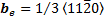, c-type screw dislocations with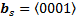and mixed a+c-type dislocations with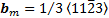. The density of edge dislocations, that are responsible for lattice twisting within the plane of the sample, exceeds the density of screw and mixed dislocations causing the lattice tilt out of the plane of the layer.

The dislocation density can be measured directly by TEM, but the accuracy is rather poor due to the limited area covered by TEM micrographs. The main significance of this technique is the possibility of determining the type of the dislocations as well as the kind of their arrangement. Alternatively, X-ray diffraction can be used to detect the lattice distortions caused by the presence of dislocations averaged over a sufficiently large sample area. The effect of lattice distortions on the diffraction peak broadening is well known and there is a vast amount of literature dealing with this topic. While the widths of the peaks can be measured by various X-ray diffraction techniques and evaluated by fitting to different types of model curves, their interpretation in terms of dislocation density is not straightforward. As regards the measuring techniques, there is an important difference between polycrystalline and single crystalline (epitaxial) samples. While in the former case a large number of diffractions can be measured within one powder diffraction pattern recorded e. g. in Bragg-Brentano set-up, measuring of (001) oriented single crystals in symmetric configuration provides only diffractions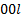that are sensitive to lattice tilt and can therefore reveal only the presence of screw (eventually screw component of mixed) TDs. The edge dislocations influence the lattice planes perpendicular to the surface and measuring the corresponding diffractions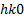requires the grazing incidence set-up.

An alternative skew geometry enabling to utilize the widths of diffractions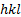that are inclined by an angle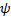with respect to surface normal was proposed by Sun et al.  and Lee et al. . Their approach is based on the model that supposes a mosaic structure of epitaxial films having a large lattice mismatch . The width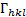of the diffractionis given as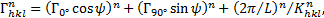(1)

where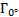and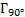are the FWHMs of the diffractionsand, respectively,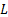is the lateral domain size and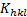is the magnitude of the diffraction vector. The exponent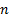varies between 1 (Lorentzian intensity profile) and 2 (Gaussian profile). The third term in (1) corresponds to the size broadening effect that in some cases has to be taken into account.

Measuring of at least one symmetric (at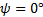) and one skew diffraction atenables one to obtain the extrapolated value ofcorresponding to twist of the mosaic blocks due to the presence of edge TDs.

In the vast majority of analyses of the quality of epitaxial layers the formulas originally derived for polycrystalline metallic samples are used for evaluation of the TDs densities from the diffraction peak widths [6-8]. Two limiting cases are distinguished: (i) random distribution of TDs, in which the dislocation density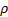is related to peak width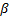as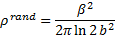(2)

and (ii) correlated distribution when the dislocations are localized at the boundaries of mosaic blocks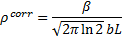(3)

where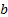is the magnitude of Burgers vector andis the average size of the blocks. It is worth noting that for polycrystalline samples the formulas (2) and (3) do not provide the possibility to distinguish between screw and edge dislocations. In order to solve this problem more sophisticated approaches are required [9, 10] that take into consideration the mutual orientation of the dislocation line, possible Burgers vectors and diffraction vectors and eventually the crystal anisotropy. These complications are considerably reduced for epitaxial layers, where only one direction of TDs and, in principle, only two types of Burgers vectors have to be considered. Setting the values ofandfor the peak widthsin (2) or (3) and using the appropriate magnitudes of Burgers vectors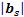and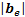, one can easily calculate the density of screw and edge dislocations. The results evidently depend on the supposed dislocation arrangement that has to be verified e. g. by TEM observation along with the estimation of the parameter.

More precise approach based on the analysis of the shape of the rocking curves was proposed by Kaganer and co-workers [11, 12]. They fit the measured intensities by the numerical Fourier transformation of the correlation function proposed by Krivoglaz  in the whole range of rocking curves including the peak tails. In addition to dislocation density, the model allows to extract also the so called cut-off radius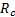. This parameter was introduced by Wilkens  in order to characterize the correlation of dislocations within the framework of “restricted random distribution” model. Its value gives the size of the domains within which the dislocation distribution is random (the sum of all Burgers vectors in the domain is zero). However, the application of this model is rather laborious and not suitable for everyday use by technologists. For practical purposes approximate formulas were derived on the basis of this model  in the forms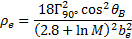(4)

for edge dislocations and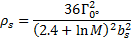(5)

for screw ones.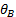is the Bragg angle of the measured diffraction and the dimensionless parameter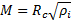depending on the corresponding TDs densities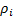varies between 1 and 2. Surprisingly, compared to (2), these equations result in four times higher edge dislocation density and an order higher screw dislocation density .

It is seen that there is a large discrepancy between different approaches to the evaluation of TDs densities. While the dependence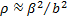for random distribution is generally accepted, the numerical factor can considerably vary, eventually depends on dislocation densities. This uncertainty can be partially excluded by comparison of the results with the TEM observation, although the precision of dislocation density measurement by TEM is rather low. Once the ratio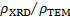is established for a given type of epitaxial system (and eventually laboratory), the calibration can be used for further XRD analyses. This procedure was applied for the evaluation of TDs in InN layers grown on GaN . The densities of both screw and edge TDs were calculated according to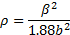(6)

where 1.88 is the calibration constant obtained from the comparison with TEM.

In this contribution the TDs density was evaluated in both layers of InN/GaN/sapphire(0001) sample. The InN and GaN layers were 0.5 μm and 0.3 μm thick, respectively. The details of the growth procedure are described elsewhere . High resolution X-ray diffraction analysis was carried out using Bruker D8 DISCOVER diffractometer equipped with X-ray tube with rotating Cu anode operating at 12 kW. The measurements were performed with parabolic Goebel mirror and four-bounce Ge 022 Bartels monochromator in the primary beam. All diffraction curves were recorded in an open detector mode. The InN layer on GaN was fully relaxed as confirmed by measuring of one asymmetricdiffraction. The density of dislocations with screw and edge components in both InN and GaN layers was evaluated from the X-ray rocking curves. FWHMs of two symmetric diffraction –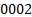,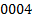and three skew ones –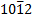,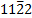and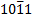were determined. Four of them are shown for InN layer in Fig. 1. Comparison of the peak widths of the same diffractions for InN and GaN are shown in Fig. 2. It is seen that for InN layer the peak broadening systematically increases with the tilting angleindicating that the density of edge TDs exceeds the density of screw ones. On the contrary, the peak widths practically do not change for GaN layer.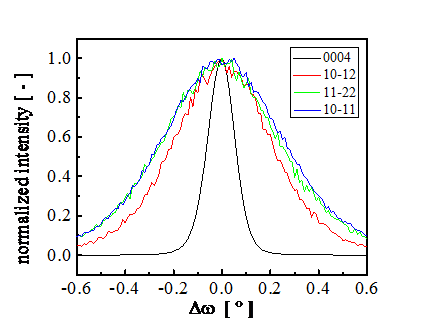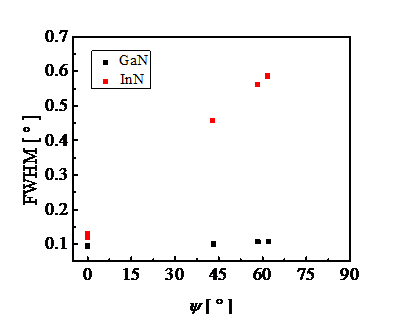###### Figure 2. Dependence of FWHMs of rocking curves on the tilting angle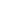.

The TDs densitiesand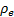were calculated according to (6), where the FWHMs of rocking curves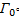and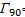at the inclination angle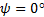and at the extrapolated value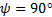were set for the parameter. Gaussian type of rocking curves was supposed, hence the exponent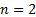was used in (1) for calculation of. The size effect (third term in (1)) was neglected in the calculation. The values of the tilting anglefor skew diffractions were calculated from the lattice parameters of InN (PDF 00-050-1239) and GaN (PDF 00-050-0792). The magnitudes of Burgers vectors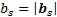and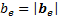are equal to the lattice parametersand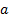, respectively,  for both hexagonal materials.

The obtained TDs densities are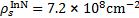,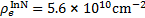,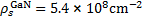and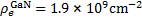. For both layers the same value 1.88 of the calibration constant was used in (6). Generally, one should be cautious with the choice of calibration proposed by different authors. The ratiomay vary significantly from 0.1 to 7 as summarized in . But in spite of this uncertainty, the increase of the density of edge TDs in the InN layer in comparison to GaN is evident. While the density of screw dislocations is practically the same, new edge TDs are generated during the growth of InN layer and their density exceeds the density of edge TDs “coming” from GaN layer by more than one order of magnitude. This is probably caused by large mismatch between the GaN and InN lattices that is ~ 10% for fully relaxed InN layer.

The analyzed samples were rather thick and the corresponding diffractions of InN and GaN were well separated even in the skew geometry used in the experiment. This is due to the large difference between the lattice parameters of these materials. However, one can encounter some difficulties when attempting to measure the TDs densities in multilayered ternary compounds having similar lattice parameters. The use of analyser crystal instead of open detector system can improve the resolution, however, this modification can seriously decrease the intensity. This is particularly important if the measurement should be performed on samples with the layer thicknesses in the range of tens of nanometers. These values are typical for up-to-date technologies. As regards the calculated TDs densities, one should reconcile oneself to the fact that the precision of density evaluation is seriously limited. This is not caused by the possible error of measurement but is rather a consequence of the fact that each model intended to describe the dislocation distribution is too far from reality.

1.       S. C. Jain, M. Willander, J. Narayan, J. Appl. Phys., 87, (2000), 965.

2.       W. V. Lundin, A. E. Nikolaev, A. V. Sakharov, S. O. Usov, E. E. Zavarin, P. N. Brunkov, M. A. Yagovkina, N. A. Cherkashin, A. F. Tsatsulnikov, Semiconductors, 48, (2014), 53.

3.       Y. J. Sun, O. Brandt, T. Y. Liu, A. Trampert, K. H. Ploog, Appl. Phys. Lett. 81, (2002), 4928.

4.       S. R. Lee, A. M. West, A. A. Allerman, K. E. Waldrip, D. M. Follstaedt, P. P. Provencio, D. D. Koleske, and C. R. Abernathy, Appl. Phys. Lett. 86, (2005), 241904.

5.       V. Srikant, J. S. Speck, D. R. Clarke, J. Appl. Phys. 82, (1997), 4286.

6.       P. Gay, P. B. Hirsch, A. Kelly, Acta Metall. 1, (1953), 315.

7.       C. G. Dunn, E. F. Koch, Acta Metall. 5, (1957), 548.

8.       R. Chierchia, T. Böttcher, H. Heinke, S. Einfeldt, S. Figge, D. Hommel, J. Appl. Phys. 93, (2003), 8918.

9.       M. A. Krivoglaz, X-ray and Neutron Diffraction in Nonideal Crystals. Berlin: Springer-Verlag, 1996.

10.    R. Kužel, Z. Kristallogr. 222, (2007), 136.

11.    V. M. Kaganer, O. Brandt, A. Trampert, and K. H. Ploog, Phys. Rev. B,72, (2005), 045423.

12.    V. S. Kopp, V. M. Kaganer, M. V. Baidakova, W. V. Lundin, A. E. Nikolaev, E. V. Verkhovtceva, M. A. Yagovkina, N. Cherkashin, J. Appl. Phys. 115, (2014), 073507.

13.    C. S. Gallinat, G. Koblmüller, Feng Wu, J. S. Speck, J. Appl. Phys. 107, (2010), 053517.

14.    Ch. Zervos, A. Adikimenakis, P. Beleniotis, A. Kostoupolos, M. Kayambaki, K. Tsagaraki, G. Konstantinidis, A. Georgakilas, Appl. Phys. Lett. 108, (2016), 142102.

The author gratefully acknowledges A. Georgakilas and A. Adikimenakis from FORTH, Greece for providing the sample.